Search by Topic

Resources tagged with Multiplication & division similar to Going Round in Circles:

Filter by: Content type:
Age range:
Challenge level:

There are 45 results

Broad Topics > Calculations and Numerical Methods > Multiplication & divisionGoing Round in Circles

Age 11 to 14 Challenge Level:

Mathematicians are always looking for efficient methods for solving problems. How efficient can you be?As Easy as 1,2,3

Age 11 to 14 Challenge Level:

When I type a sequence of letters my calculator gives the product of all the numbers in the corresponding memories. What numbers should I store so that when I type 'ONE' it returns 1, and when I type. . . .Skeleton

Age 11 to 14 Challenge Level:

Amazing as it may seem the three fives remaining in the following `skeleton' are sufficient to reconstruct the entire long division sum.A One in Seven Chance

Age 11 to 14 Challenge Level:

What is the remainder when 2^{164}is divided by 7?Oh! Hidden Inside?

Age 11 to 14 Challenge Level:

Find the number which has 8 divisors, such that the product of the divisors is 331776.Age 11 to 14 Challenge Level:

Powers of numbers behave in surprising ways. Take a look at some of these and try to explain why they are true.Largest Number

Age 11 to 14 Challenge Level:

What is the largest number you can make using the three digits 2, 3 and 4 in any way you like, using any operations you like? You can only use each digit once.Method in Multiplying Madness?

Age 7 to 14 Challenge Level:

Watch our videos of multiplication methods that you may not have met before. Can you make sense of them?Powerful Factorial

Age 11 to 14 Challenge Level:

6! = 6 x 5 x 4 x 3 x 2 x 1. The highest power of 2 that divides exactly into 6! is 4 since (6!) / (2^4 ) = 45. What is the highest power of two that divides exactly into 100!?Eminit

Age 11 to 14 Challenge Level:

The number 8888...88M9999...99 is divisible by 7 and it starts with the digit 8 repeated 50 times and ends with the digit 9 repeated 50 times. What is the value of the digit M?3388

Age 11 to 14 Challenge Level:

Using some or all of the operations of addition, subtraction, multiplication and division and using the digits 3, 3, 8 and 8 each once and only once make an expression equal to 24.Factoring Factorials

Age 11 to 14 Challenge Level:

Find the highest power of 11 that will divide into 1000! exactly.Slippy Numbers

Age 11 to 14 Challenge Level:

The number 10112359550561797752808988764044943820224719 is called a 'slippy number' because, when the last digit 9 is moved to the front, the new number produced is the slippy number multiplied by 9.X Marks the Spot

Age 11 to 14 Challenge Level:

When the number x 1 x x x is multiplied by 417 this gives the answer 9 x x x 0 5 7. Find the missing digits, each of which is represented by an "x" .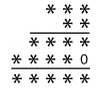Long Multiplication

Age 11 to 14 Challenge Level:

A 3 digit number is multiplied by a 2 digit number and the calculation is written out as shown with a digit in place of each of the *'s. Complete the whole multiplication sum.Remainders

Age 7 to 14 Challenge Level:

I'm thinking of a number. My number is both a multiple of 5 and a multiple of 6. What could my number be?Times Right

Age 11 to 16 Challenge Level:

Using the digits 1, 2, 3, 4, 5, 6, 7 and 8, mulitply a two two digit numbers are multiplied to give a four digit number, so that the expression is correct. How many different solutions can you find?Factorial

Age 14 to 16 Challenge Level:

How many zeros are there at the end of the number which is the product of first hundred positive integers?Expenses

Age 14 to 16 Challenge Level:

What is the largest number which, when divided into 1905, 2587, 3951, 7020 and 8725 in turn, leaves the same remainder each time?Repeaters

Age 11 to 14 Challenge Level:

Choose any 3 digits and make a 6 digit number by repeating the 3 digits in the same order (e.g. 594594). Explain why whatever digits you choose the number will always be divisible by 7, 11 and 13.Diggits

Age 11 to 14 Challenge Level:

Can you find what the last two digits of the number $4^{1999}$ are?Two Many

Age 11 to 14 Challenge Level:

What is the least square number which commences with six two's?Age 11 to 14 Challenge Level:

Visitors to Earth from the distant planet of Zub-Zorna were amazed when they found out that when the digits in this multiplication were reversed, the answer was the same! Find a way to explain. . . .Round and Round and Round

Age 11 to 14 Challenge Level:

Where will the point stop after it has turned through 30 000 degrees? I took out my calculator and typed 30 000 ÷ 360. How did this help?Like Powers

Age 11 to 14 Challenge Level:

Investigate $1^n + 19^n + 20^n + 51^n + 57^n + 80^n + 82^n$ and $2^n + 12^n + 31^n + 40^n + 69^n + 71^n + 85^n$ for different values of n.Thirty Six Exactly

Age 11 to 14 Challenge Level:

The number 12 = 2^2 � 3 has 6 factors. What is the smallest natural number with exactly 36 factors?Multiply the Addition Square

Age 11 to 14 Challenge Level:

If you take a three by three square on a 1-10 addition square and multiply the diagonally opposite numbers together, what is the difference between these products. Why?A First Product Sudoku

Age 11 to 14 Challenge Level:

Given the products of adjacent cells, can you complete this Sudoku?More Mods

Age 14 to 16 Challenge Level:

What is the units digit for the number 123^(456) ?Missing Multipliers

Age 7 to 14 Challenge Level:

What is the smallest number of answers you need to reveal in order to work out the missing headers?Multiples Sudoku

Age 11 to 14 Challenge Level:

Each clue in this Sudoku is the product of the two numbers in adjacent cells.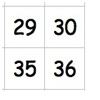So It's Times!

Age 7 to 14 Challenge Level:

How will you decide which way of flipping over and/or turning the grid will give you the highest total?Number Rules - OK

Age 14 to 16 Challenge Level:

Can you convince me of each of the following: If a square number is multiplied by a square number the product is ALWAYS a square number...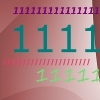Ones Only

Age 11 to 14 Challenge Level:

Find the smallest whole number which, when mutiplied by 7, gives a product consisting entirely of ones.Magic Potting Sheds

Age 11 to 14 Challenge Level:

Mr McGregor has a magic potting shed. Overnight, the number of plants in it doubles. He'd like to put the same number of plants in each of three gardens, planting one garden each day. Can he do it?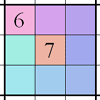Integrated Product Sudoku

Age 11 to 16 Challenge Level:

This Sudoku puzzle can be solved with the help of small clue-numbers on the border lines between pairs of neighbouring squares of the grid.The Remainders Game

Age 7 to 14 Challenge Level:

Play this game and see if you can figure out the computer's chosen number.Countdown

Age 7 to 14 Challenge Level:

Here is a chance to play a version of the classic Countdown Game.One O Five

Age 11 to 14 Challenge Level:

You can work out the number someone else is thinking of as follows. Ask a friend to think of any natural number less than 100. Then ask them to tell you the remainders when this number is divided by. . . .2010: A Year of Investigations

Age 5 to 14

This article for teachers suggests ideas for activities built around 10 and 2010.More Magic Potting Sheds

Age 11 to 14 Challenge Level:

The number of plants in Mr McGregor's magic potting shed increases overnight. He'd like to put the same number of plants in each of his gardens, planting one garden each day. How can he do it?A Chance to Win?

Age 11 to 14 Challenge Level:

Imagine you were given the chance to win some money... and imagine you had nothing to lose...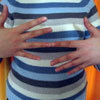What Is the Question?

Age 11 to 16 Challenge Level:

These pictures and answers leave the viewer with the problem "What is the Question". Can you give the question and how the answer follows?Galley Division

Age 14 to 16 Challenge Level:

Can you explain how Galley Division works?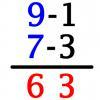Vedic Sutra - All from 9 and Last from 10

Age 14 to 16 Challenge Level:

Vedic Sutra is one of many ancient Indian sutras which involves a cross subtraction method. Can you give a good explanation of WHY it works?